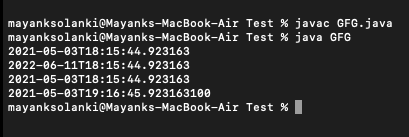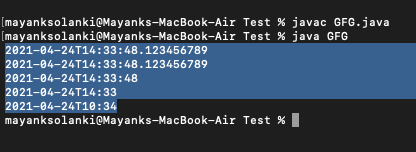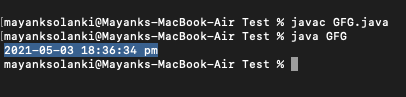Open in App
Not now

# java.time.LocalDateTime Class in Java

• Last Updated : 26 May, 2021

java.time.LocalDateTime class, introduced in Java 8, represents a local date-time object without timezone information. The LocalDateTime class in Java is an immutable date-time object that represents a date in the yyyy-MM-dd-HH-mm-ss.zzz format. It implements the ChronoLocalDateTime interface and inherits the object class.

Wherever we need to represent time without a timezone reference, we can use the LocalDateTime instances. LocalDateTime, for example, can be used to start batch jobs in any application. Jobs will be run at a fixed time in the timezone in which the server is located. Note LocalDateTime instances are immutable and thread.

Syntax: Class declaration

public final class LocalDateTime

extends Object

Methods of this class are as follows:

Some more methods to modify local time are as follows  in LocalDateTime can be used to get to a new localdatetime instance relative to an existing localdatetime instance. They are namely as follows:

plusYears(), plusMonths(), plusDays(), plusHours(), plusMinutes(), plusSeconds(), plusNanos(), minusYears(), minusMonths(), minusDays(), minusHours(), minusMinutes(), minusSeconds(), minusNanos()

Examples 1:

## Java

 `// Java Program to illustrate LocalDateTime Class of java.time package ` `// Importing LocalDateTime class from java.time package``import` `java.time.LocalDateTime;` `// Main class for LocalDateTime``public` `class` `GFG {` `    ``// Main driver method``    ``public` `static` `void` `main(String[] args)``    ``{``        ``// Creating an object of LocalDateTime class``        ``// in the main() method``        ``LocalDateTime now = LocalDateTime.now();` `        ``// Print statement``        ``System.out.println(now);` `        ``// Adding 1 year, 1 month, 1 week and 1 day``        ``LocalDateTime localDateTime1 = now.plusYears(``1``)``                                           ``.plusMonths(``1``)``                                           ``.plusWeeks(``1``)``                                           ``.plusDays(``1``);``        ``// Print statement``        ``System.out.println(localDateTime1);` `        ``// Subtracting 1 year, 1 month, 1 week and 1 day``        ``LocalDateTime localDateTime2``            ``= localDateTime1.minusYears(``1``)``                  ``.minusMonths(``1``)``                  ``.minusWeeks(``1``)``                  ``.minusDays(``1``);``        ``// Print statement``        ``System.out.println(localDateTime2);` `        ``// Adding 1 hour, 1 minute, 1 second and 100``        ``// nanoseconds``        ``LocalDateTime localDateTime3``            ``= localDateTime2.plusHours(``1``)``                  ``.plusMinutes(``1``)``                  ``.plusSeconds(``1``)``                  ``.plusNanos(``100``);``        ``// Print statement``        ``System.out.println(localDateTime3);` `        ``// Subtracting 1 hour, 1 minute, 1 second and 100``        ``// nanoseconds``        ``LocalDateTime localDateTime4``            ``= localDateTime3.minusHours(``1``)``                  ``.minusMinutes(``1``)``                  ``.minusSeconds(``1``)``                  ``.minusNanos(``100``);``        ``// Print statement``        ``System.out.println(localDateTime4);``    ``}``}`

Output:Example 2: Creating a specified time

## Java

 `// Java Program to illustrate LocalDateTime Class``// of java.time package by creating specific time` `// Importing required classes from resp packages``import` `java.time.*;``import` `java.time.format.*;` `// main class``class` `GFG {` `    ``// Main driver method``    ``public` `static` `void` `main(String[] args)``    ``{` `        ``// Milliseconds``        ``LocalDateTime localDateTime1 = LocalDateTime.of(``            ``2021``, ``04``, ``24``, ``14``, ``33``, ``48``, ``123456789``);``        ``// Print statement``        ``System.out.println(localDateTime1);` `        ``// Month``        ``LocalDateTime localDateTime2 = LocalDateTime.of(``            ``2021``, Month.APRIL, ``24``, ``14``, ``33``, ``48``, ``123456789``);``        ``// Print statement``        ``System.out.println(localDateTime2);` `        ``// Seconds``        ``LocalDateTime localDateTime3 = LocalDateTime.of(``            ``2021``, Month.APRIL, ``24``, ``14``, ``33``, ``48``);``        ``// Print statement``        ``System.out.println(localDateTime3);` `        ``// Minutes``        ``LocalDateTime localDateTime4 = LocalDateTime.of(``            ``2021``, Month.APRIL, ``24``, ``14``, ``33``);``        ``// Print statement``        ``System.out.println(localDateTime4);` `        ``// Local date + Local time``        ``LocalDate date = LocalDate.of(``2021``, ``04``, ``24``);``        ``LocalTime time = LocalTime.of(``10``, ``34``);` `        ``LocalDateTime localDateTime5``            ``= LocalDateTime.of(date, time);``        ``// Print statement``        ``System.out.println(localDateTime5);``    ``}``}`

Output:Example 3: Format LocalDateTime to string

To format a local time to the desired string representation, use the LocalDateTime.format(DateTimeFormatter) method.

## Java

 `// Java Program to illustrate LocalDateTime Class by``// Formatting LocalDateTime to string` `// Importing all classes from java.time package``import` `java.time.LocalDateTime;``import` `java.time.format.*;``import` `java.util.*;` `// Main class``class` `GFG {` `    ``// Main driver method``    ``public` `static` `void` `main(String[] args)``    ``{` `        ``// Creating an object of DateTimeFormatter class``        ``DateTimeFormatter formatter``            ``= DateTimeFormatter.ofPattern(``                ``"yyyy-MM-dd HH:mm:ss a"``);` `        ``// Creating an object of LocalDateTime class``        ``// and getting local date and time using now()``        ``// method``        ``LocalDateTime now = LocalDateTime.now();` `        ``// Formatting LocalDateTime to string``        ``String dateTimeString = now.format(formatter);` `        ``// Print and Display``        ``System.out.println(dateTimeString);``    ``}``}`

Output:Note: In order to parse a string to LocalDateTime, convert time in a string to a local time instance, the LocalDateTime class has two overloaded parse() methods.

• parse(CharSequence text)
• parse(CharSequence text, DateTimeFormatter formatter)

My Personal Notes arrow_drop_up